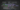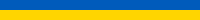# First Class Functions in PythonApr 15, 2019

Categories
Hack
Learning
Tags
Programming
Python
Do you like our work......we hire!

Never miss our publications about Open Source, big data and distributed systems, low frequency of one email every two months.

I recently watched a talk by Dave Cheney about first class functions in Go. Python supports first class functions too, so can we use them in the same ways? Absolutely.

I have been using Python for a while now. It makes for a nice complement to Bash when I need to incorporate somewhat complex logic into scripts. It often makes for very clean and readable code when manipulating simple data.

When learning to use a language, I find it important to see what can be done using only the language’s innate features. For instance, learning how to use — and not overuse — list comprehensions is a crucial requirement to mastering Python. This article explores what can be done with first class functions in Python while keeping the code clean.

First, what does “Python supports first class functions” mean? Simply put, functions can be used as if they were values. You can store them in variables, pass them to other functions as arguments, etc.

Dave Cheney used a simple calculator as an example, so let’s start with that. Here’s one way of implementing it:

``````class Calculator:

def __init__(self):
self.num = 0.0

def get(self):
return self.num

self.num += n

def subtract(self, n):
self.num -= n

def multiply(self, n):
self.num *= n``````

Familiarity with Python — or virtually any object-oriented language — is all it takes to understand the code above. Here’s how it can be used:

``````calc = Calculator()

calc.multiply(5)
calc.subtract(3)

print(calc.get())  # 7.0``````

Now that the calculator works, this code can be imported into any script that might need it. But, what if the script needs to use division? The calculator does not implement that yet.

That capability could be added easily by writing a new method for the Calculator class. Although, what if someone later needs to compute a logarithm? Or factorials? In an ideal world, this calculator should be able to do any kind of numerical computation without needing to have its code edited.

This is where first class functions come in. Remember the definition from earlier: functions can be stored in variables and passed as arguments to other functions.

Here is some code to show how functions can be stored in variables:

``````def rand():
return 4  # chosen by fair dice roll (https://xkcd.com/221/)

print(rand())  # 4
print(rand)    # <function rand at 0x7fe0d1a37048>

also_rand = rand
print(also_rand())  # 4
print(also_rand)    # <function rand at 0x7fe0d1a37048>

"""My suggestion just do
def rand():
return 4  # chosen by fair dice roll (https://xkcd.com/221/)
type(rand) # function
also_rand = rand
also_rand() == rand() # True
id(rand) == id(randd) # True
"""``````

In the code above, rand is actually a variable of the ‘function’ type. Notice that also_rand has the same exact value as rand, name and all.

Functions can also be passed as arguments to other functions, like this:

``````def hello():
print("Hi there!")

def call_three_times(any_func):
any_func()
any_func()
any_func()

call_three_times(hello)  # Hi there!
# Hi there!
# Hi there!``````

This will remind many Python developers of functions like map and filter that take functions as arguments, just like call_three_times above. This is called functional programming.

Back to the calculator. Users should be able to pass any function to the calculator and have it run that function. Here is how it could be coded:

``````class Calculator:

def __init__(self):
self.num = 0.0

def get(self):
return self.num

def do(self, operation):
self.num = operation(self.num)``````

The code is much shorter now, which is normal: the calculator can do anything but it does not know how to actually do any of it. Here are some operations for it to run:

``````def add_two(n):
return n + 2

def multiply_by_three(n):
return n * 3

calc = Calculator()

calc.do(multiply_by_three)

print(calc.get())  # 6.0``````

All of this works, but it does not make for very flexible code. There is an entire function just to add 2. If one wanted to add 5 next, there would need to be an add_five function. This will get old very quickly.

This is a good use case for lambdas. They allow creating short anonymous functions, i.e. functions that are not explicitly defined with def. They can be used to rewrite the code above like this:

``````calc = Calculator()

calc.do(lambda x: x + 2)
calc.do(lambda x: x + 5)
calc.do(lambda x: x * 3)

print(calc.get())  # 21.0``````

While not having to define functions for every operation the calculator should run is nice, there is such a thing as too many lambdas: the code above is not very easy to read. If someone wanted to know what the code above did, they would spend a lot of time reading those lambdas in order to understand exactly what it is they are doing. This code needs to be made more readable.

What if those lambdas could be replaced by functions with explicit names? Kind of like add_two from earlier only with more re-usability. We could write a function that returns a lambda.

``````def add(n):
return lambda x: x + n

def multiply(n):
return lambda x: x * n

calc = Calculator()

calc.do(multiply(5))

print(calc.get())  # 13.0``````

In the code above, add and multiply are functions that return a function. The returned function is then called by the calculator. This is what functional programming is all about and makes for very readable code.

Lambdas can only use one expression, which makes them great for simple things like addition but insufficient when it comes to more complex logic. Lambdas are not all we can use, however. Any function can be returned.

``````def add(n):
def anonymous(x):
return x + n
return anonymous

def gcd(n):
def helper(a, b):
if b == 0:
return a
r = a % b
return helper(b, r)
def anonymous(x):
return helper(x, n)
return anonymous

calc = Calculator()

calc.do(gcd(42))

print(calc.get())  # 14.0``````

It is clear now that the calculator can apply any behavior encoded inside a function. The only prerequisite is to respect a simple contract: the function passed to the calculator’s do method must take one value as an argument and return one value. This means that any function that already matches these criteria can be sent to the calculator, including functions from other libraries. For example:

``````import math

return lambda x: x + n

calc = Calculator()

calc.do(math.sqrt)

print(calc.get())  # 3.872983346207417``````

Support for first class functions is no small feature. While using functions in this way may seem intimidating at first, one gets the hang of it pretty quickly.

If there is only one thing to take away from this article, it is that functions can be used to build other functions. While it might seem complicated, it can actually make code much easier to read and understand. It can also allow writing complex — and even configurable — logic that abides by very simple contracts.

The Go community makes extensive use of all this in their code, and it is serving them well. Maybe some of these patterns can be used in Python code as well.